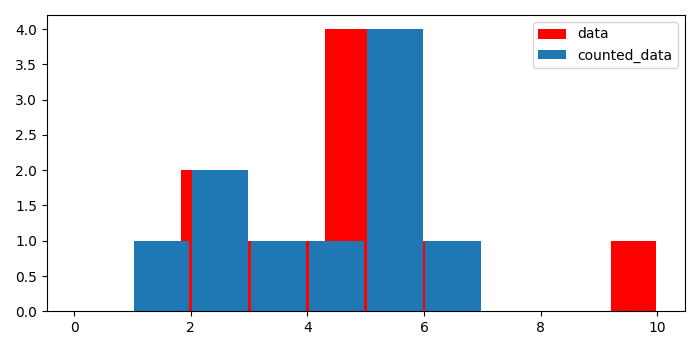# Plotting a histogram from pre-counted data in Matplotlib

To plot a histogram from pre-counted data in matplotlib, we can take the following steps −

• Create a list of numbers.

• Make a pre-counted list with the help of input data.

• Plot a histogram with data, color=red, and label=data, using hist() method.

• Plot another histogram with counted data, color=default, and label=counted_data, using hist() method.

• To place the legend, use legend() method.

• To display the figure, use show() method.

## Example

from matplotlib import pyplot as plt
plt.rcParams["figure.figsize"] = [7.00, 3.50]
plt.rcParams["figure.autolayout"] = True
data = [1, 2, 2, 3, 4, 5, 5, 5, 5, 6, 10]
counted_data = {1: 1, 2: 2, 3: 1, 4: 1, 5: 4, 6: 1, 10: 1}
hh = plt.hist(data, bins=len(data), rwidth=.95, color='red', label="data")
hh1 = plt.hist(counted_data.keys(), weights=counted_data.values(),
bins=range(10), rwidth=.95, label="counted_data")
plt.legend()
plt.show()

## Output